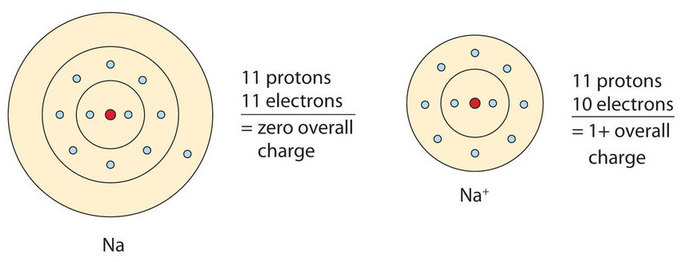# 3.1: Ions

$$\newcommand{\vecs}{\overset { \rightharpoonup} {\mathbf{#1}} }$$ $$\newcommand{\vecd}{\overset{-\!-\!\rightharpoonup}{\vphantom{a}\smash {#1}}}$$$$\newcommand{\id}{\mathrm{id}}$$ $$\newcommand{\Span}{\mathrm{span}}$$ $$\newcommand{\kernel}{\mathrm{null}\,}$$ $$\newcommand{\range}{\mathrm{range}\,}$$ $$\newcommand{\RealPart}{\mathrm{Re}}$$ $$\newcommand{\ImaginaryPart}{\mathrm{Im}}$$ $$\newcommand{\Argument}{\mathrm{Arg}}$$ $$\newcommand{\norm}{\| #1 \|}$$ $$\newcommand{\inner}{\langle #1, #2 \rangle}$$ $$\newcommand{\Span}{\mathrm{span}}$$ $$\newcommand{\id}{\mathrm{id}}$$ $$\newcommand{\Span}{\mathrm{span}}$$ $$\newcommand{\kernel}{\mathrm{null}\,}$$ $$\newcommand{\range}{\mathrm{range}\,}$$ $$\newcommand{\RealPart}{\mathrm{Re}}$$ $$\newcommand{\ImaginaryPart}{\mathrm{Im}}$$ $$\newcommand{\Argument}{\mathrm{Arg}}$$ $$\newcommand{\norm}{\| #1 \|}$$ $$\newcommand{\inner}{\langle #1, #2 \rangle}$$ $$\newcommand{\Span}{\mathrm{span}}$$

Learning Objectives

• Distinguish the difference between the two types of ions.
• Describe ion formation using electron configurations.

## Ions

As introduced in Chapter 2, atoms contain a nucleus with neutrons and positively charged protons, surrounded by negatively charged electrons. In an atom, the total number of electrons, negative charge, equals the total number of protons, positive charge, and therefore, atoms are electrically neutral or uncharged. If an atom loses or gains electrons, it will become a positively or negatively charged particle, called an ion. The loss of one or more electrons results in more protons than electrons and an overall positively charged ion, called a cation. For example, a sodium atom with one less electron is a cation, Na+, with a +1 charge (Figure $$\PageIndex{1}$$).Figure $$\PageIndex{1}$$: On the left, a sodium atom has 11 electrons. On the right, the sodium ion only has 10 electrons and a 1+ charge.

When an atom gains one or more electrons, it becomes a negatively charged anion, because there are more electrons than protons. When chlorine gains one electron it forms a chloride ion, Cl, with a –1 charge (Figures $$\PageIndex{2}$$)

The names for positive and negative ions are pronounced CAT-eye-ons (cations) and ANN-eye-ons (anions), respectively.Figure $$\PageIndex{2}$$: The Formation of a Chlorine Ion. On the left, the chlorine atom has 17 electrons. On the right, the chloride ion has 18 electrons and has a 1− charge.

## Electron Transfer

Ions are formed when an atom, usually on the left side of the periodic table, reacts with and transfers one or more electrons to another atom, usually on the right side of the periodic table. These electrons are usually lost from and gained into the valence shell, or outermost energy level. Therefore, it is useful to look at electron configurations to further illustrate ion formation and electron transfer between atoms.

The electron configuration for sodium shows that there are ten core electrons and one valence electron in the third energy level. When sodium loses the single valence electron, forming the cation Na+, the electron configuration is now identical to that of neon, a stable noble gas.

$\begin{array}{lcl} \ce{Na} & \rightarrow & \ce{Na^+} + \ce{e^-} \\ 1s^2 \: 2s^2 \: 2p^6 \: 3s^1 & & 1s^2 \: 2s^2 \: 2p^6 \end{array}$

Chlorine also has ten core electrons and valence electrons in the third energy level. However, chlorine has seven valence electrons, one less than the noble gas argon, which has eight valence electrons. Thus, chlorine will gain one electron, forming the anion, Cl, and achieving a stable noble gas configuration.

$\begin{array}{lcl} \ce{Cl} + \ce{e^-} & \rightarrow & \ce{Cl^-} \\ 1s^2 \: 2s^2 \:2p^6 \: 3s^2 \: 3p^5 & & 1s^2 \: 2s^2 \: 2p^6 \: 3s^2 \: 3p^6 \end{array}$

Cations are named using the element name plus "ion" to indicate it is charged. Anions are named by changing the element name ending to "ide". For example, a magnesium ion is formed when neutral magnesium loses electrons and a fluoride ion is formed when neutral fluorine gains electrons.

The resulting, oppositely charged cation and anion (sodium and chloride ions) are attracted to one another, due to electrostatic interactions, and thus form an ionic compound, called sodium chloride. Notice that there are no leftover electrons in this electron transfer reaction. The number of electrons lost by the sodium atom (one) equals the number of electrons gained by the chlorine atom (one), so the compound is electrically neutral. In macroscopic samples of sodium chloride, there are billions and billions of sodium and chloride ions, although there is always the same number of cations and anions.

Example $$\PageIndex{1}$$

Write the electron configuration of aluminum atom (Z = 13) and underline the valence electrons. How many electrons are gained/lost to form an aluminum ion, Al3+? Write the electron configuration for this ion.

Solution

The electron configuration of Al atom is 1s22s22p63s23p1. Aluminum has three valence electrons in the third energy level, (3s23p1). The cation, Al3+, is formed when these three valence electrons are lost, leaving the configuration for the noble gas neon, 1s22s22p6.

Exercise $$\PageIndex{1}$$

Write the electron configuration of oxygen atom (= 8) and underline the valence electrons. How many electrons are gained/lost to form an oxide ion, O2–? Write the electron configuration for this ion.

The electron configuration of O atom is 1s22s22p4. Oxygen has six valence electrons in the second energy level, (2s22p4). The anion O2 is formed when two electrons are gained in the valence shell. The resulting electron configuration, 1s22s22p6, is also identical to the configuration for the noble gas neon.

## Key Takeaways

• Ions can be positively charged or negatively charged.

## Contributors

• Lisa Sharpe Elles, University of Kansas

## Exercises

1. What are the two types of ions?

2. When the following atoms become ions, what charges do they acquire?

1. Li
2. S
3. Ca
4. F

4. Identify each as a cation, an anion, or neither.

1. H+
2. Cl
3. O2
4. Ba2+
5. CH4
6. CS2

5. Identify each as a cation, an anion, or neither.

1. NH3
2. Br
3. H
4. Hg2+
5. CCl4
6. SO3

6. Write the electron configuration for each ion.

1. Li+
2. Mg2+
3. F
4. S2−

7. Write the electron configuration for each ion.

1. Na+
2. Be2+
3. Cl
4. O2−

1. Cations (positive charged) and anions (negative charged)

2.

1. 1+
2. 2−
3. 2+
4. 1−

4.

1. cation
2. anion
3. neither
4. cation
5. neither
6. neither
5.
1. neither
2. anion
3. anion
4. cation
5. neither
6. neither

6.

1. 1s2
2. 1s22s22p6
3. 1s22s22p6
4. 1s22s22p63s23p6
7.
1. 1s22s22p6
2. 1s2
3. 1s22s22p63s23p6
4. 1s22s22p6

8.
1.b.c.d.9.10.11.12.13.14. 1+

15. 2+

16. 2−

17. 1−

3.1: Ions is shared under a not declared license and was authored, remixed, and/or curated by LibreTexts.